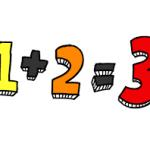Rate:1+2=3

How fast can you add and subtract? Are you smarter than a 1st grader? This is a very simple math game which all the questions & answers are combined by 12, 3, plus & minus.

Rating: 4.0909090909091 out of 5 based on 11 user ratings

1+2=3 GamePlay:

How fast can you add and subtract? Are you smarter than a 1st grader? This is a very simple math game which all the questions & answers are combined by 12, 3, plus & minus.

• Tags: